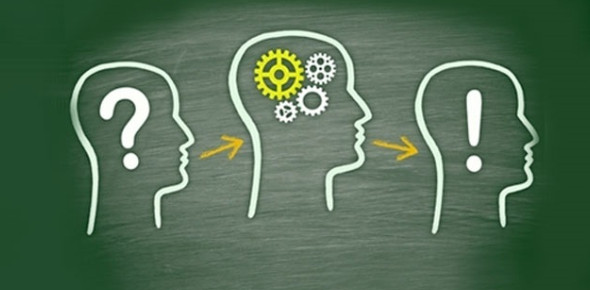SSC CGL Exam Tier-I Exam Quiz: Reasoning!

19 Questions | Total Attempts: 401Settings• 1.
Which color is opposite to yellow?
• A.

Violet

• B.

Red

• C.

Purple

• D.

Blue

• 2.
Which color is opposite to Green?
• A.

Indigo

• B.

Blue

• C.

Violet

• D.

Orange

• 3.
Two positions of a dice are shown below. When 3 is at the bottom, what number will be at the top? Question Figures
• A.

6

• B.

3

• C.

4

• D.

2

• 4.
Two positions of a dice are shown below: When the heart shape is at the top, what will be at the bottom?
• A.
• B.
• C.
• D.
• 5.
Study the two different positions of a dice. When the face containing one dot is at the bottom then how many dots would be there on the top face?
• A.

6

• B.

5

• C.

4

• D.

3

• 6.
Two positions of a dice are shown below. When the number 'one' is on the top, what number will be at the bottom? Question Figures
• A.
• B.
• C.
• D.
• 7.
Two positions of a dice are shown below: When 3 is at the bottom, which number is at the top?
• A.

4

• B.

5

• C.

2

• D.

1

• 8.
Two positions of a dice are shown below: When '2' is at the bottom, what number will be at the top?
• A.

3

• B.

5

• C.

1

• D.

6

• 9.
Three positions of a cube are given. Based on them find out which number is found opposite of number 2 in a given cube?
• A.

2

• B.

5

• C.

1

• D.

6

• 10.
Study the two different positions of a cube given below with dots from 1 to 6 marked on its faces. Find out how many dots are contained on the face opposite to that containing 4 dots.
• A.

1

• B.

2

• C.

3

• D.

5

• 11.
Study the two different positions of a cube given below with dots from 1 to 6 marked on its faces. Find out how many dots are contained on the face opposite to that containing 3 dots.
• A.

6

• B.

2

• C.

1

• D.

5

• 12.
Given below are four views of a cube. Each face is marked with a certain symbol. In figure B which symbol will appear on the face opposite to the face having symbol ∗? Question Figures:
• A.
• B.
• C.
• D.
• 13.
Four views of one cube are given below. Each of the six faces of cube is numbered. In figure 3 which number will be opposite to the face with number 3?
• A.

1

• B.

2

• C.

5

• D.

6

• 14.
Choose from the four answer figures the figure that will be formed when question figure is folded into a box. Question Figure
• A.